# Carnegie Mellon 1 Carnegie Mellon ThreadLevel Parallelism 14

• Slides: 56Carnegie Mellon 1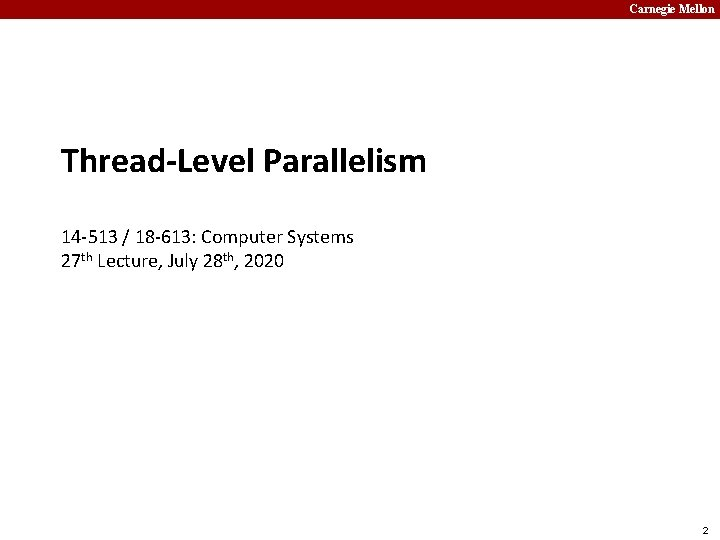Carnegie Mellon Thread-Level Parallelism 14 -513 / 18 -613: Computer Systems 27 th Lecture, July 28 th, 2020 2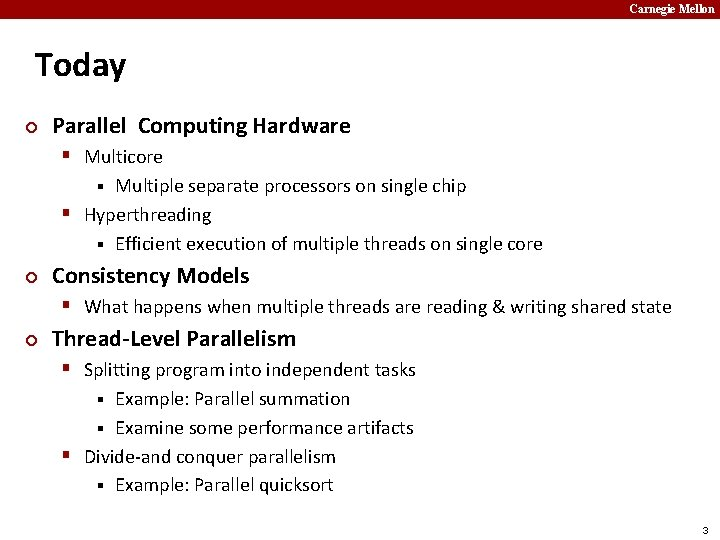Carnegie Mellon Today ¢ Parallel Computing Hardware § Multicore Multiple separate processors on single chip § Hyperthreading § Efficient execution of multiple threads on single core § ¢ Consistency Models § What happens when multiple threads are reading & writing shared state ¢ Thread-Level Parallelism § Splitting program into independent tasks Example: Parallel summation § Examine some performance artifacts § Divide-and conquer parallelism § Example: Parallel quicksort § 3Carnegie Mellon Typical Multicore Processor Core 0 Core n-1 Regs L 1 d-cache L 1 i-cache L 2 unified cache … L 1 d-cache L 1 i-cache L 2 unified cache L 3 unified cache (shared by all cores) Main memory ¢ Multiple processors operating with coherent view of memory 4Carnegie Mellon Out-of-Order Processor Structure Instruction Control Instruction Decoder Registers Op. Queue Instruction Cache PC Functional Units Int Arith ¢ ¢ Int Arith FP Arith Load / Store Data Cache Instruction control dynamically converts program into stream of operations Operations mapped onto functional units to execute in parallel 5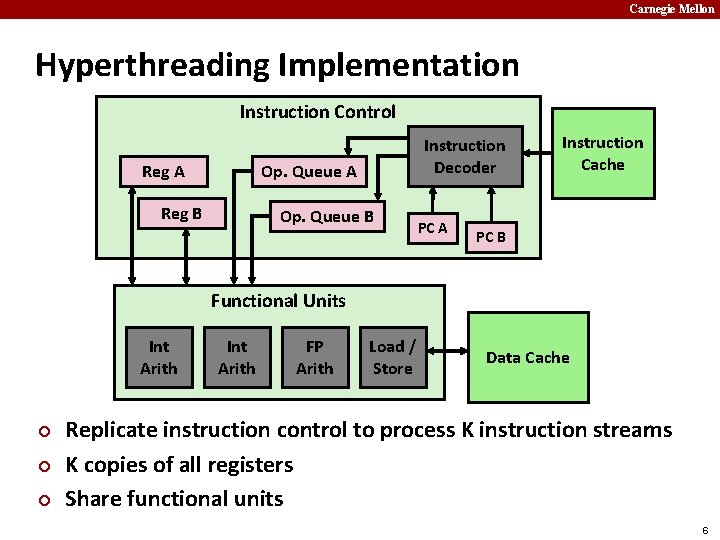Carnegie Mellon Hyperthreading Implementation Instruction Control Reg A Instruction Decoder Op. Queue A Reg B Op. Queue B PC A Instruction Cache PC B Functional Units Int Arith ¢ ¢ ¢ Int Arith FP Arith Load / Store Data Cache Replicate instruction control to process K instruction streams K copies of all registers Share functional units 6Carnegie Mellon Benchmark Machine ¢ ¢ Get data about machine from /proc/cpuinfo Shark Machines § § Intel Xeon E 5520 @ 2. 27 GHz Nehalem, ca. 2010 8 Cores Each can do 2 x hyperthreading 7Carnegie Mellon Exploiting parallel execution ¢ ¢ So far, we’ve used threads to deal with I/O delays § e. g. , one thread per client to prevent one from delaying another Multi-core CPUs offer another opportunity § Spread work over threads executing in parallel on N cores § Happens automatically, if many independent tasks § e. g. , running many applications or serving many clients § Can also write code to make one big task go faster § ¢ by organizing it as multiple parallel sub-tasks Shark machines can execute 16 threads at once § 8 cores, each with 2 -way hyperthreading § Theoretical speedup of 16 X § never achieved in our benchmarks 8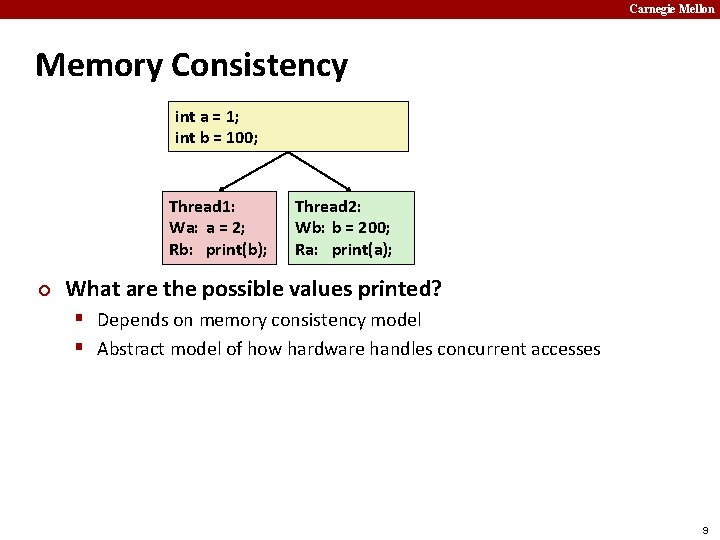Carnegie Mellon Memory Consistency int a = 1; int b = 100; Thread 1: Wa: a = 2; Rb: print(b); ¢ Thread 2: Wb: b = 200; Ra: print(a); What are the possible values printed? § Depends on memory consistency model § Abstract model of how hardware handles concurrent accesses 9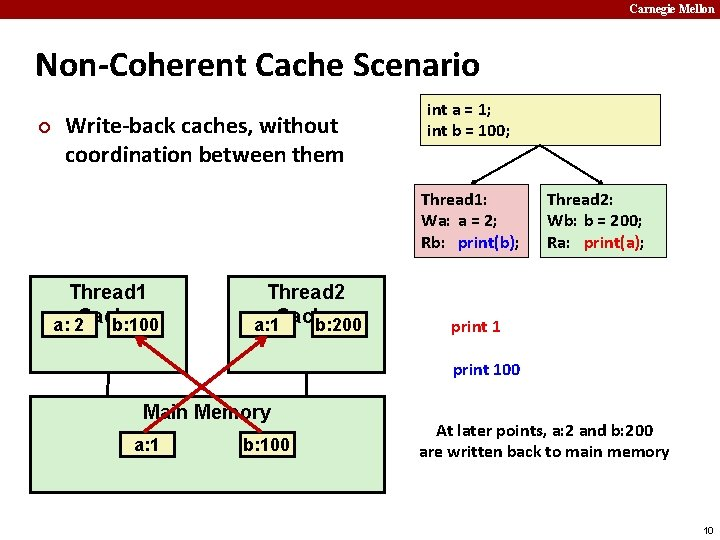Carnegie Mellon Non-Coherent Cache Scenario ¢ Write-back caches, without coordination between them int a = 1; int b = 100; Thread 1: Wa: a = 2; Rb: print(b); Thread 1 a: 2 Cache b: 100 Thread 2 a: 1 Cache b: 200 Thread 2: Wb: b = 200; Ra: print(a); print 100 Main Memory a: 1 b: 100 At later points, a: 2 and b: 200 are written back to main memory 10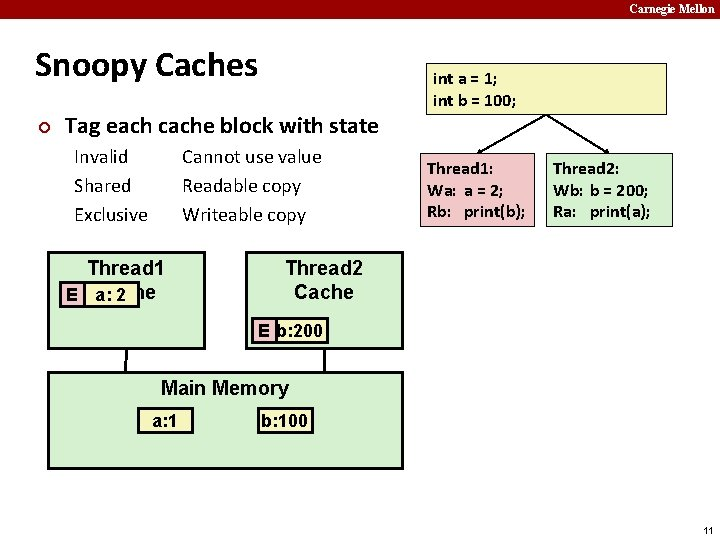Carnegie Mellon Snoopy Caches ¢ Tag each cache block with state Invalid Shared Exclusive Cannot use value Readable copy Writeable copy Thread 1 Cache E a: 2 int a = 1; int b = 100; Thread 1: Wa: a = 2; Rb: print(b); Thread 2: Wb: b = 200; Ra: print(a); Thread 2 Cache E b: 200 Main Memory a: 1 b: 100 11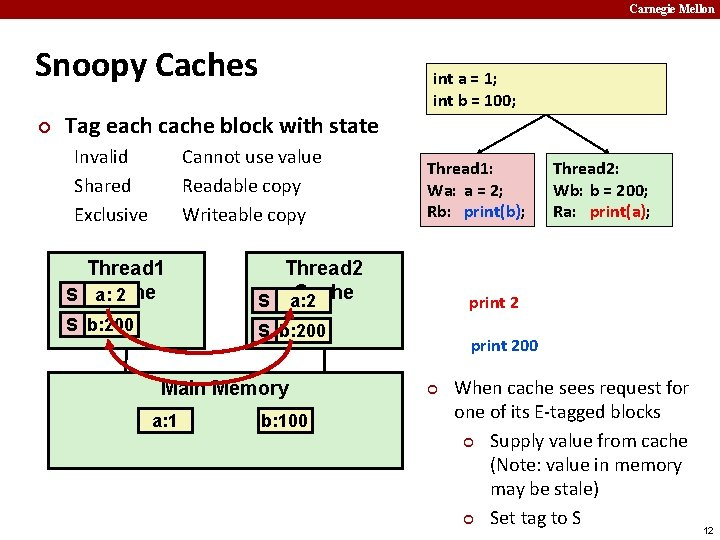Carnegie Mellon Snoopy Caches ¢ Tag each cache block with state Invalid Shared Exclusive Cannot use value Readable copy Writeable copy Thread 1 Cache E a: S 2 Thread 2 Cache S a: 2 S b: 200 E b: 200 S b: 200 Main Memory a: 1 b: 100 int a = 1; int b = 100; Thread 1: Wa: a = 2; Rb: print(b); Thread 2: Wb: b = 200; Ra: print(a); print 200 ¢ When cache sees request for one of its E-tagged blocks ¢ Supply value from cache (Note: value in memory may be stale) ¢ Set tag to S 12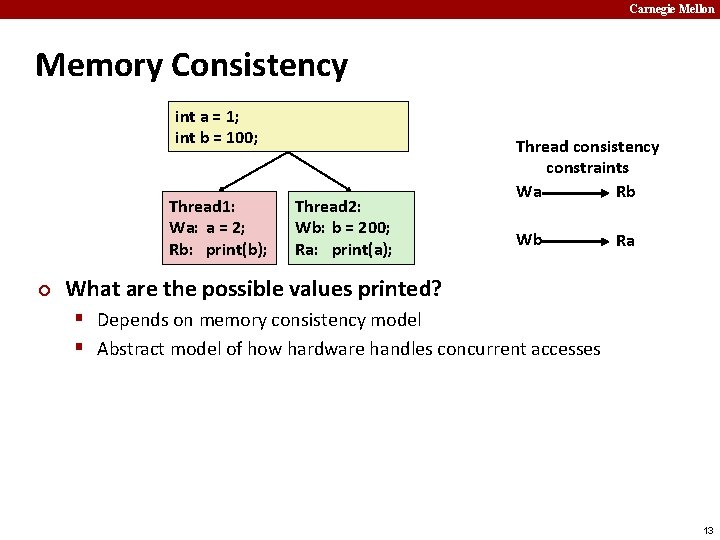Carnegie Mellon Memory Consistency int a = 1; int b = 100; Thread 1: Wa: a = 2; Rb: print(b); ¢ Thread 2: Wb: b = 200; Ra: print(a); Thread consistency constraints Wa Rb Wb Ra What are the possible values printed? § Depends on memory consistency model § Abstract model of how hardware handles concurrent accesses 13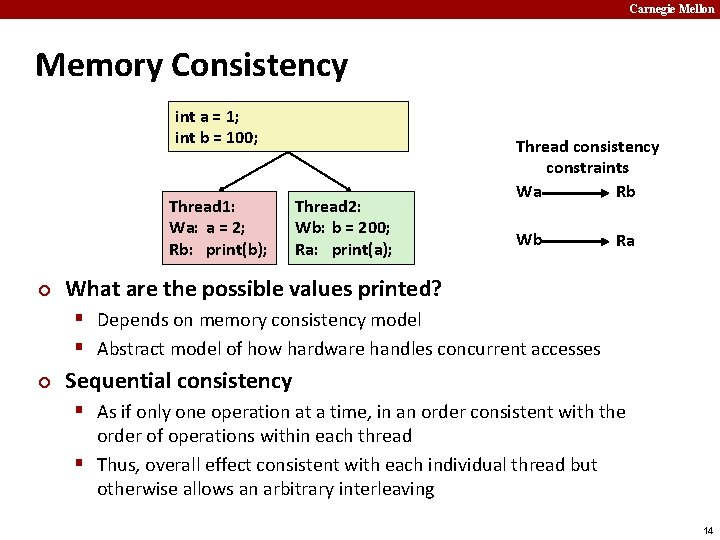Carnegie Mellon Memory Consistency int a = 1; int b = 100; Thread 1: Wa: a = 2; Rb: print(b); ¢ Thread 2: Wb: b = 200; Ra: print(a); Thread consistency constraints Wa Rb Wb Ra What are the possible values printed? § Depends on memory consistency model § Abstract model of how hardware handles concurrent accesses ¢ Sequential consistency § As if only one operation at a time, in an order consistent with the order of operations within each thread § Thus, overall effect consistent with each individual thread but otherwise allows an arbitrary interleaving 14Carnegie Mellon Sequential Consistency Example Thread consistency constraints Wa Rb int a = 1; int b = 100; Thread 1: Wa: a = 2; Rb: print(b); Wb Thread 2: Wb: b = 200; Ra: print(a); Rb Wa Wb Ra Wb ¢ Impossible outputs Wa Ra Wb Ra 100, 2 Rb Ra 200, 2 Ra Wa Rb 2, 200 Rb 1, 200 Ra Rb 2, 200 Rb Ra 200, 2 § 100, 1 and 1, 100 § Would require reaching both Ra and Rb before either Wa or Wb 15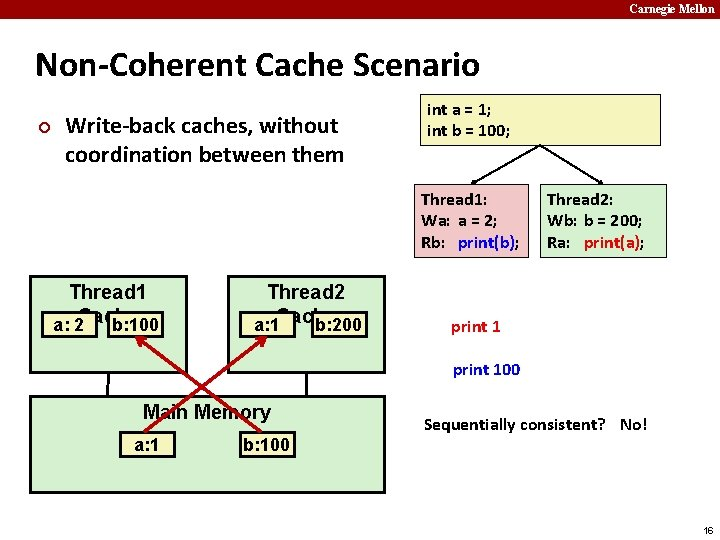Carnegie Mellon Non-Coherent Cache Scenario ¢ Write-back caches, without coordination between them int a = 1; int b = 100; Thread 1: Wa: a = 2; Rb: print(b); Thread 1 a: 2 Cache b: 100 Thread 2 a: 1 Cache b: 200 Thread 2: Wb: b = 200; Ra: print(a); print 100 Main Memory a: 1 b: 100 Sequentially consistent? No! 16Carnegie Mellon Non-Sequentially Consistent Scenario ¢ Coherent caches, but thread consistency constraints violated due to operation reordering a: 2 b: 200 Thread 1 Cache b: 100 int a = 1; int b = 100; Thread 1: 3 Wa: a = 2; 2 Rb: print(b); Thread 2: Wb: b = 200; Ra: print(a); 4 1 Thread 2 Cache a: 1 print 100 Main Memory a: 1 ¢ b: 100 Arch lets reads finish before writes b/c single thread accesses different memory locations 17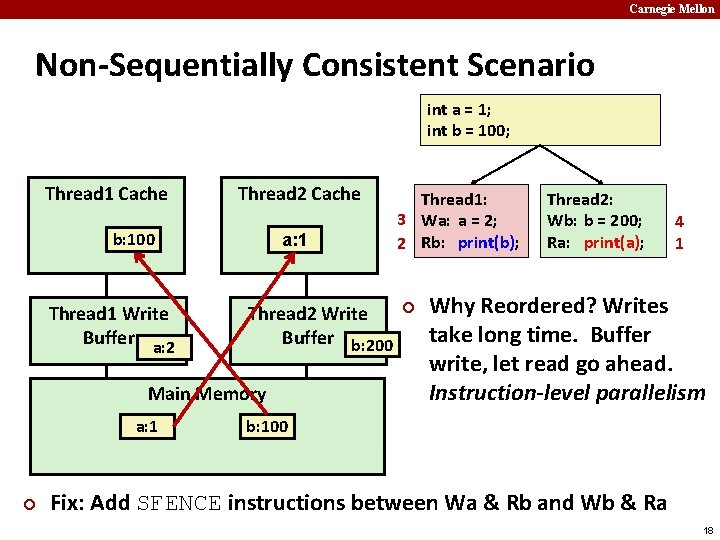Carnegie Mellon Non-Sequentially Consistent Scenario int a = 1; int b = 100; Thread 1 Cache Thread 2 Cache b: 100 Thread 1 Write Buffer a: 2 a: 1 Thread 2 Write Buffer b: 200 Main Memory a: 1 ¢ Thread 1: 3 Wa: a = 2; 2 Rb: print(b); ¢ Thread 2: Wb: b = 200; Ra: print(a); 4 1 Why Reordered? Writes take long time. Buffer write, let read go ahead. Instruction-level parallelism b: 100 Fix: Add SFENCE instructions between Wa & Rb and Wb & Ra 18Carnegie Mellon Memory Models ¢ Sequentially Consistent: § Each thread executes in proper order, any interleaving ¢ To ensure, requires § Proper cache/memory behavior § Proper intra-thread ordering constraints 19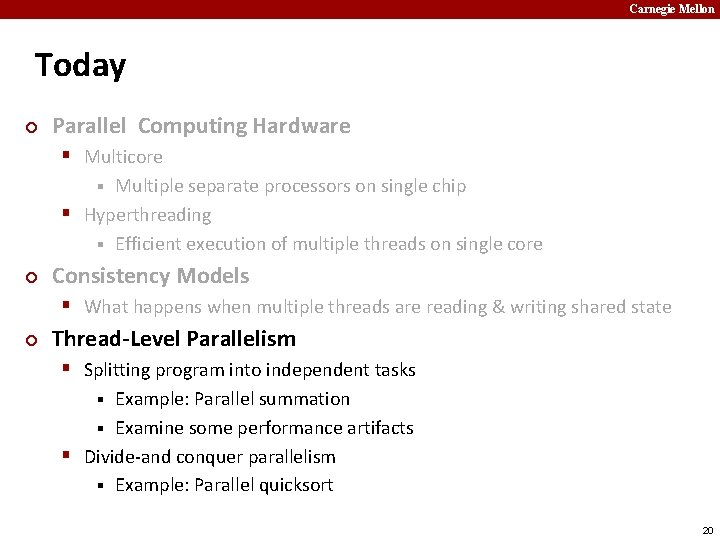Carnegie Mellon Today ¢ Parallel Computing Hardware § Multicore Multiple separate processors on single chip § Hyperthreading § Efficient execution of multiple threads on single core § ¢ Consistency Models § What happens when multiple threads are reading & writing shared state ¢ Thread-Level Parallelism § Splitting program into independent tasks Example: Parallel summation § Examine some performance artifacts § Divide-and conquer parallelism § Example: Parallel quicksort § 20Carnegie Mellon Summation Example ¢ Sum numbers 0, …, N-1 § Should add up to (N-1)*N/2 ¢ Partition into K ranges § N/K values each § Each of the t threads processes 1 range § Accumulate leftover values serially ¢ Method #1: All threads update single global variable § 1 A: No synchronization § 1 B: Synchronize with pthread semaphore § 1 C: Synchronize with pthread mutex § “Binary” semaphore. Only values 0 & 1 21Carnegie Mellon Accumulating in Single Global Variable: Declarations typedef unsigned long data_t; /* Single accumulator */ volatile data_t global_sum; /* Mutex & semaphore for global sum */ sem_t semaphore; pthread_mutex_t mutex; /* Number of elements summed by each thread */ size_t nelems_per_thread; /* Keep track of thread IDs */ pthread_t tid[MAXTHREADS]; /* Identify each thread */ int myid[MAXTHREADS]; 22Carnegie Mellon Accumulating in Single Global Variable: Declarations typedef unsigned long data_t; /* Single accumulator */ volatile data_t global_sum; /* Mutex & semaphore for global sum */ sem_t semaphore; pthread_mutex_t mutex; /* Number of elements summed by each thread */ size_t nelems_per_thread; /* Keep track of thread IDs */ pthread_t tid[MAXTHREADS]; /* Identify each thread */ int myid[MAXTHREADS]; 23Carnegie Mellon Accumulating in Single Global Variable: Declarations typedef unsigned long data_t; /* Single accumulator */ volatile data_t global_sum; /* Mutex & semaphore for global sum */ sem_t semaphore; pthread_mutex_t mutex; /* Number of elements summed by each thread */ size_t nelems_per_thread; /* Keep track of thread IDs */ pthread_t tid[MAXTHREADS]; /* Identify each thread */ int myid[MAXTHREADS]; 24Carnegie Mellon Accumulating in Single Global Variable: Operation nelems_per_thread = nelems / nthreads; /* Set global value */ global_sum = 0; Thread ID Thread routine /* Create threads and wait for them to finish */ for (i = 0; i < nthreads; i++) { myid[i] = i; Pthread_create(&tid[i], NULL, thread_fun, &myid[i]); } for (i = 0; i < nthreads; i++) Thread arguments Pthread_join(tid[i], NULL); (void *p) result = global_sum; /* Add leftover elements */ for (e = nthreads * nelems_per_thread; e < nelems; e++) result += e; 25Carnegie Mellon Thread Function: No Synchronization void *sum_race(void *vargp) { int myid = *((int *)vargp); size_t start = myid * nelems_per_thread; size_t end = start + nelems_per_thread; size_t i; for (i = start; i < end; i++) { global_sum += i; } return NULL; } 26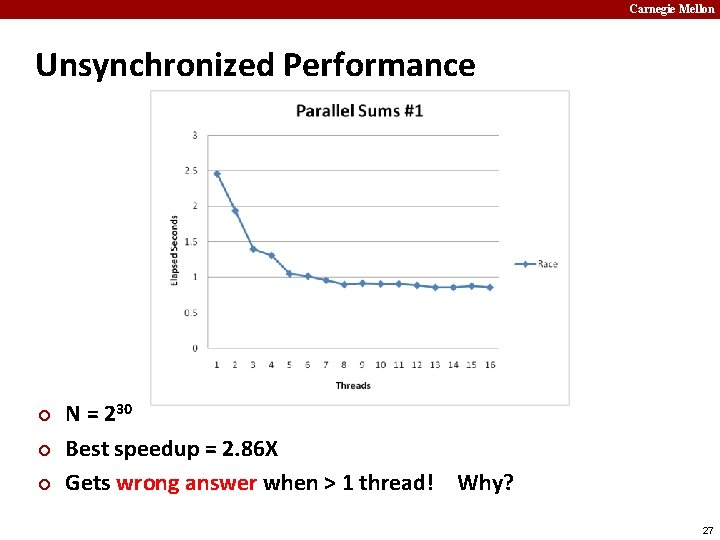Carnegie Mellon Unsynchronized Performance ¢ ¢ ¢ N = 230 Best speedup = 2. 86 X Gets wrong answer when > 1 thread! Why? 27Carnegie Mellon Thread Function: Semaphore / Mutex Semaphore void *sum_sem(void *vargp) { int myid = *((int *)vargp); size_t start = myid * nelems_per_thread; size_t end = start + nelems_per_thread; size_t i; for (i = start; i < end; i++) { sem_wait(&semaphore); global_sum += += i; i; global_sum sem_post(&semaphore); } return NULL; } Mutex pthread_mutex_lock(&mutex); global_sum += i; pthread_mutex_unlock(&mutex); 28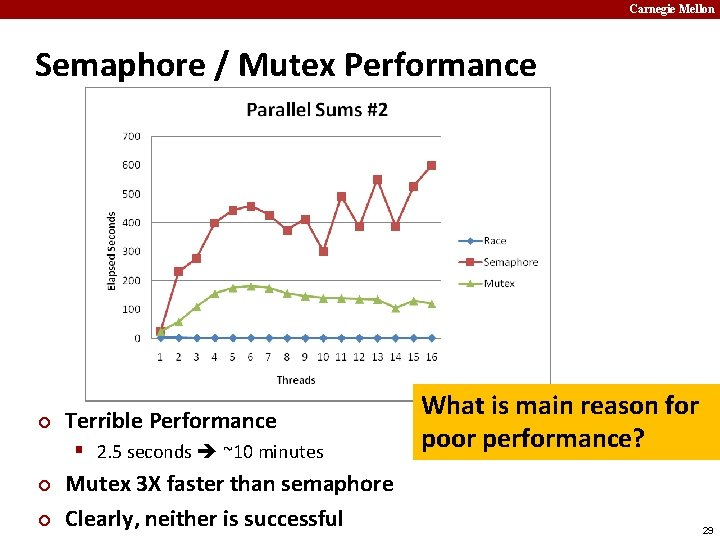Carnegie Mellon Semaphore / Mutex Performance ¢ Terrible Performance § 2. 5 seconds ~10 minutes ¢ ¢ Mutex 3 X faster than semaphore Clearly, neither is successful What is main reason for poor performance? 29Carnegie Mellon Separate Accumulation ¢ Method #2: Each thread accumulates into separate variable § 2 A: Accumulate in contiguous array elements § 2 B: Accumulate in spaced-apart array elements § 2 C: Accumulate in registers /* Partial sum computed by each thread */ data_t psum[MAXTHREADS*MAXSPACING]; /* Spacing between accumulators */ size_t spacing = 1; 30Carnegie Mellon Separate Accumulation: Operation nelems_per_thread = nelems / nthreads; /* Create threads and wait for them to finish */ for (i = 0; i < nthreads; i++) { myid[i] = i; psum[i*spacing] = 0; Pthread_create(&tid[i], NULL, thread_fun, &myid[i]); } for (i = 0; i < nthreads; i++) Pthread_join(tid[i], NULL); result = 0; /* Add up the partial sums computed by each thread */ for (i = 0; i < nthreads; i++) result += psum[i*spacing]; /* Add leftover elements */ for (e = nthreads * nelems_per_thread; e < nelems; e++) result += e; 31Carnegie Mellon Thread Function: Memory Accumulation Where is the mutex? void *sum_global(void *vargp) { int myid = *((int *)vargp); size_t start = myid * nelems_per_thread; size_t end = start + nelems_per_thread; size_t index = myid*spacing; psum[index] = 0; for (i = start; i < end; i++) { psum[index] += i; } return NULL; } 32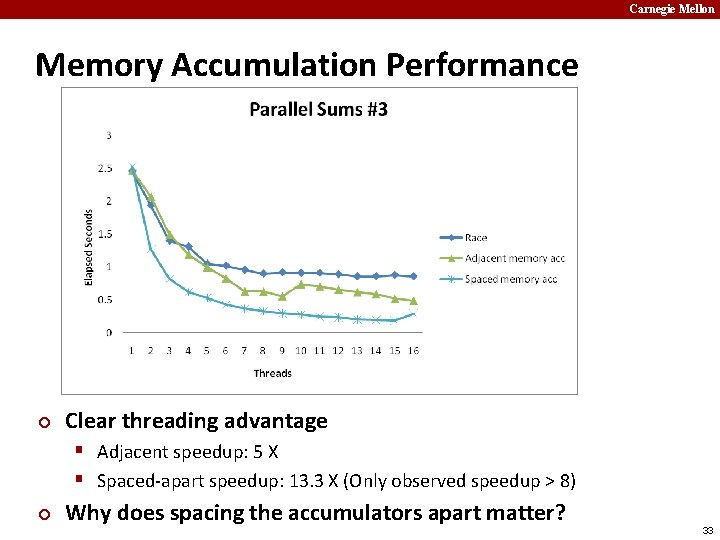Carnegie Mellon Memory Accumulation Performance ¢ Clear threading advantage § Adjacent speedup: 5 X § Spaced-apart speedup: 13. 3 X (Only observed speedup > 8) ¢ Why does spacing the accumulators apart matter? 33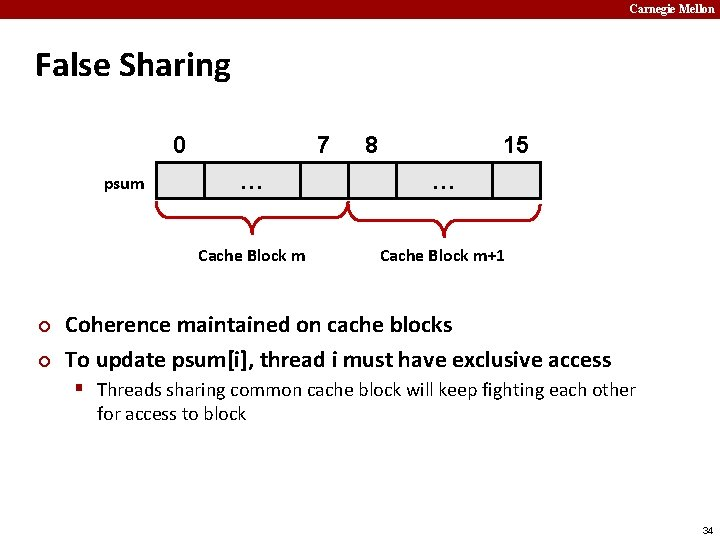Carnegie Mellon False Sharing 0 psum ¢ ¢ 7 8 15 … … Cache Block m+1 Coherence maintained on cache blocks To update psum[i], thread i must have exclusive access § Threads sharing common cache block will keep fighting each other for access to block 34Carnegie Mellon False Sharing Performance § Best spaced-apart performance 2. 8 X better than best adjacent ¢ Demonstrates cache block size = 64 § 8 -byte values § No benefit increasing spacing beyond 8 35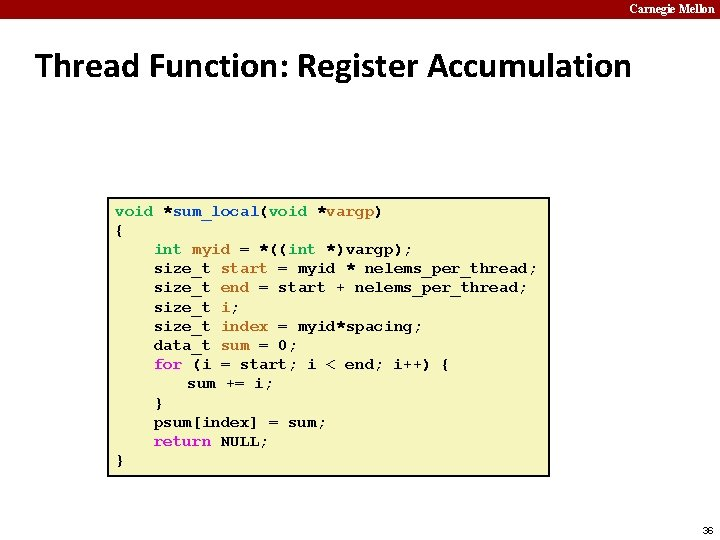Carnegie Mellon Thread Function: Register Accumulation void *sum_local(void *vargp) { int myid = *((int *)vargp); size_t start = myid * nelems_per_thread; size_t end = start + nelems_per_thread; size_t index = myid*spacing; data_t sum = 0; for (i = start; i < end; i++) { sum += i; } psum[index] = sum; return NULL; } 36Carnegie Mellon Register Accumulation Performance ¢ Clear threading advantage § Speedup = 7. 5 X ¢ Beware the speedup metric! 2 X better than fastest memory accumulation 37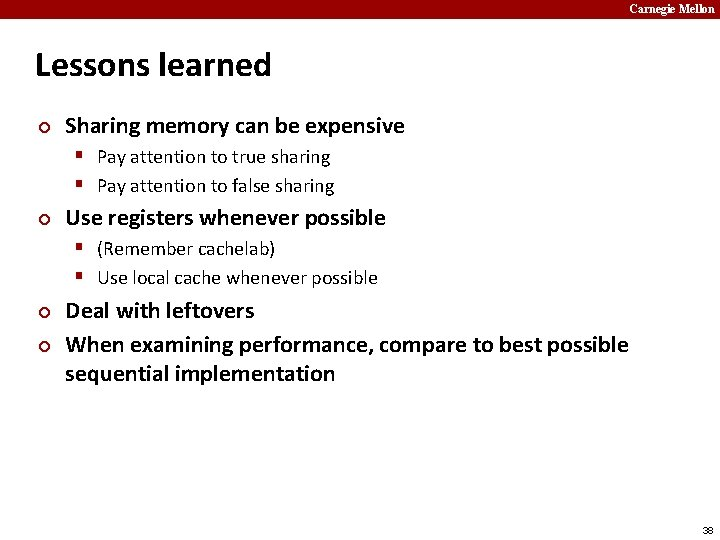Carnegie Mellon Lessons learned ¢ Sharing memory can be expensive § Pay attention to true sharing § Pay attention to false sharing ¢ Use registers whenever possible § (Remember cachelab) § Use local cache whenever possible ¢ ¢ Deal with leftovers When examining performance, compare to best possible sequential implementation 38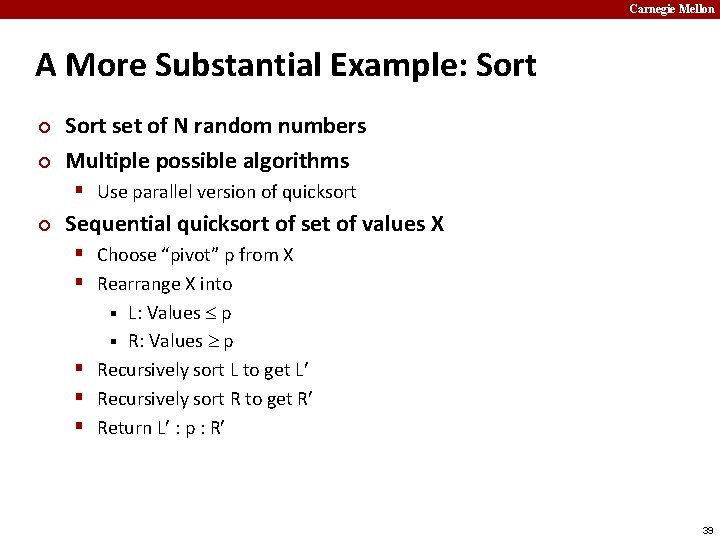Carnegie Mellon A More Substantial Example: Sort ¢ ¢ Sort set of N random numbers Multiple possible algorithms § Use parallel version of quicksort ¢ Sequential quicksort of set of values X § Choose “pivot” p from X § Rearrange X into L: Values p § Recursively sort L to get L § Recursively sort R to get R § Return L : p : R § 39Carnegie Mellon Sequential Quicksort Visualized X p L p R p 2 L 2 p 2 R 2 L 40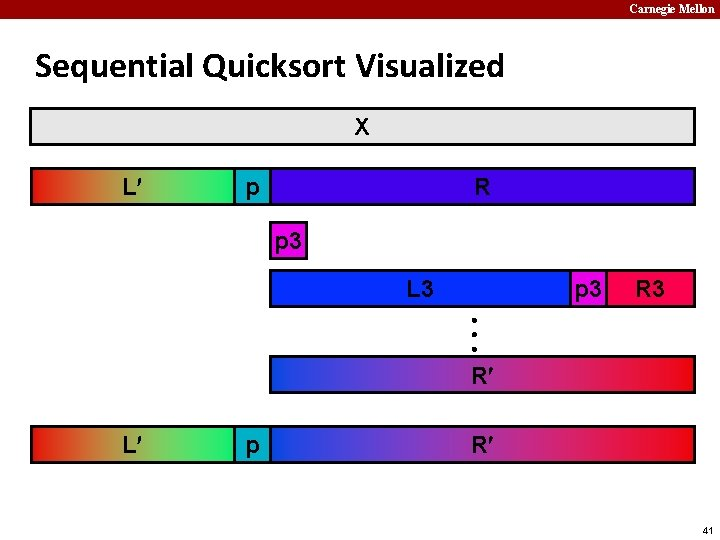Carnegie Mellon Sequential Quicksort Visualized X L p R p 3 L 3 p 3 R 3 R L p R 41Carnegie Mellon Sequential Quicksort Code void qsort_serial(data_t *base, size_t nele) { if (nele <= 1) return; if (nele == 2) { if (base > base) swap(base, base+1); return; } /* Partition returns index of pivot */ size_t m = partition(base, nele); if (m > 1) qsort_serial(base, m); if (nele-1 > m+1) qsort_serial(base+m+1, nele-m-1); } ¢ Sort nele elements starting at base § Recursively sort L or R if has more than one element 42Carnegie Mellon Parallel Quicksort ¢ Parallel quicksort of set of values X § If N Nthresh, do sequential quicksort § Else Choose “pivot” p from X § Rearrange X into – L: Values p – R: Values p § Recursively spawn separate threads – Sort L to get L – Sort R to get R § Return L : p : R § 43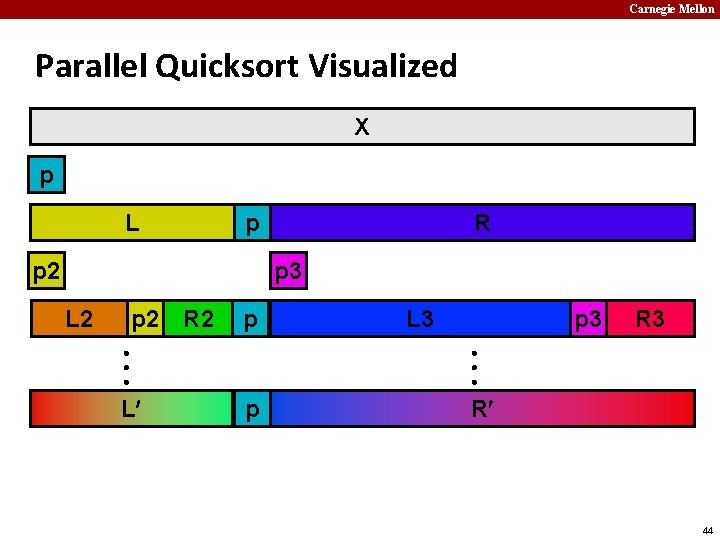Carnegie Mellon Parallel Quicksort Visualized X p L p p 2 R p 3 L 2 p 2 R 2 p L L 3 p 3 R 3 p R 44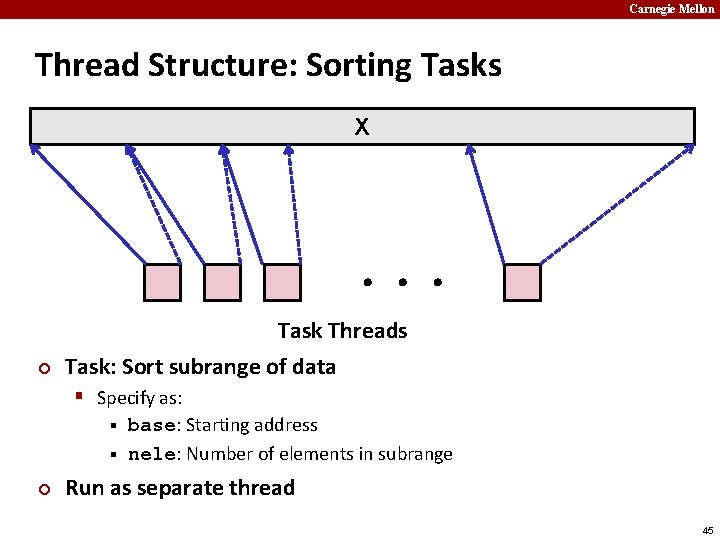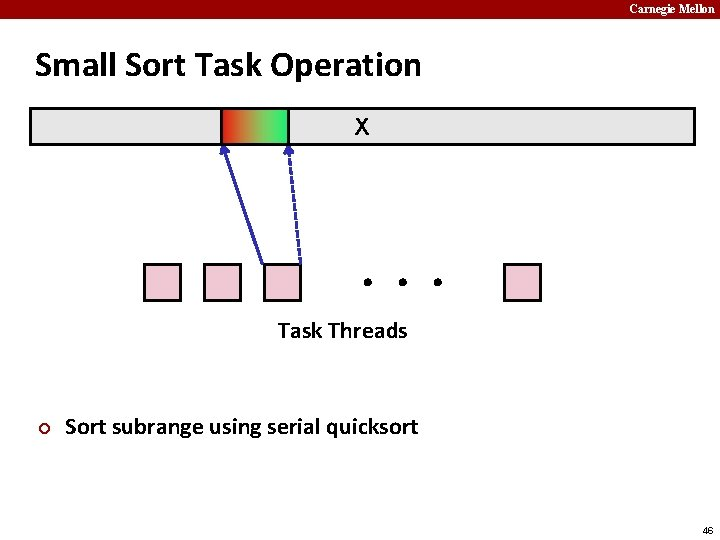Carnegie Mellon Large Sort Task Operation L p R X Partition Subrange L p R X Spawn 2 tasks 47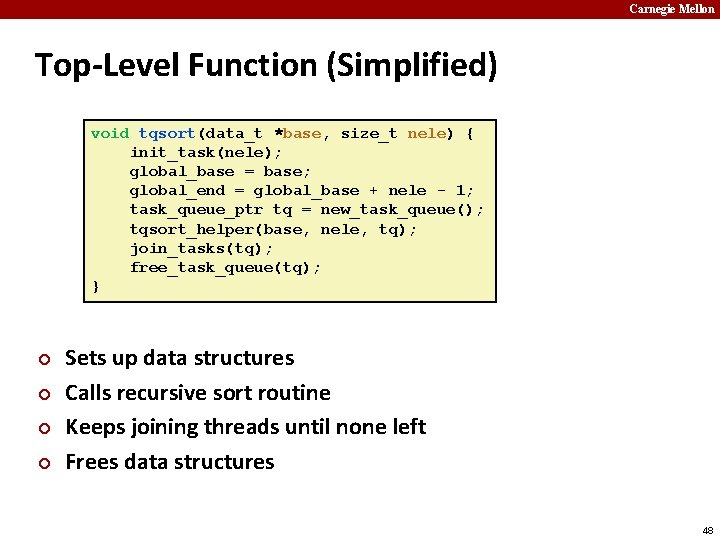Carnegie Mellon Top-Level Function (Simplified) void tqsort(data_t *base, size_t nele) { init_task(nele); global_base = base; global_end = global_base + nele - 1; task_queue_ptr tq = new_task_queue(); tqsort_helper(base, nele, tq); join_tasks(tq); free_task_queue(tq); } ¢ ¢ Sets up data structures Calls recursive sort routine Keeps joining threads until none left Frees data structures 48Carnegie Mellon Recursive sort routine (Simplified) /* Multi-threaded quicksort */ static void tqsort_helper(data_t *base, size_t nele, task_queue_ptr tq) { if (nele <= nele_max_sort_serial) { /* Use sequential sort */ qsort_serial(base, nele); return; } sort_task_t *t = new_task(base, nele, tq); spawn_task(tq, sort_thread, (void *) t); } ¢ ¢ Small partition: Sort serially Large partition: Spawn new sort task 49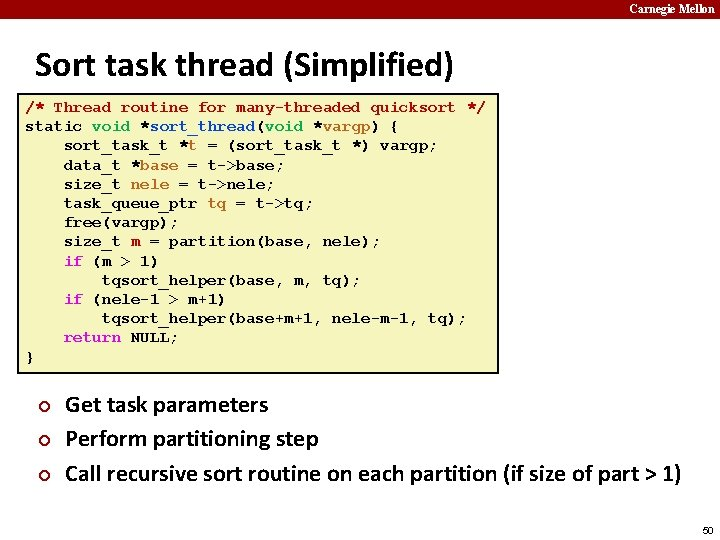Carnegie Mellon Sort task thread (Simplified) /* Thread routine for many-threaded quicksort */ static void *sort_thread(void *vargp) { sort_task_t *t = (sort_task_t *) vargp; data_t *base = t->base; size_t nele = t->nele; task_queue_ptr tq = t->tq; free(vargp); size_t m = partition(base, nele); if (m > 1) tqsort_helper(base, m, tq); if (nele-1 > m+1) tqsort_helper(base+m+1, nele-m-1, tq); return NULL; } ¢ ¢ ¢ Get task parameters Perform partitioning step Call recursive sort routine on each partition (if size of part > 1) 50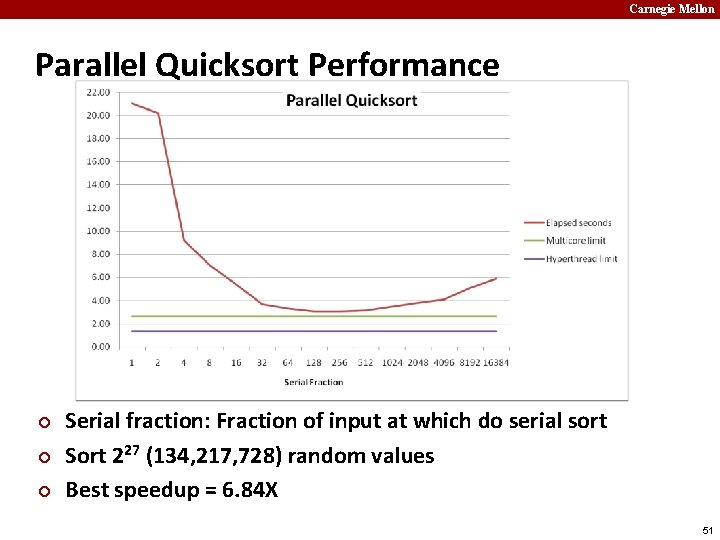Carnegie Mellon Parallel Quicksort Performance ¢ ¢ ¢ Serial fraction: Fraction of input at which do serial sort Sort 227 (134, 217, 728) random values Best speedup = 6. 84 X 51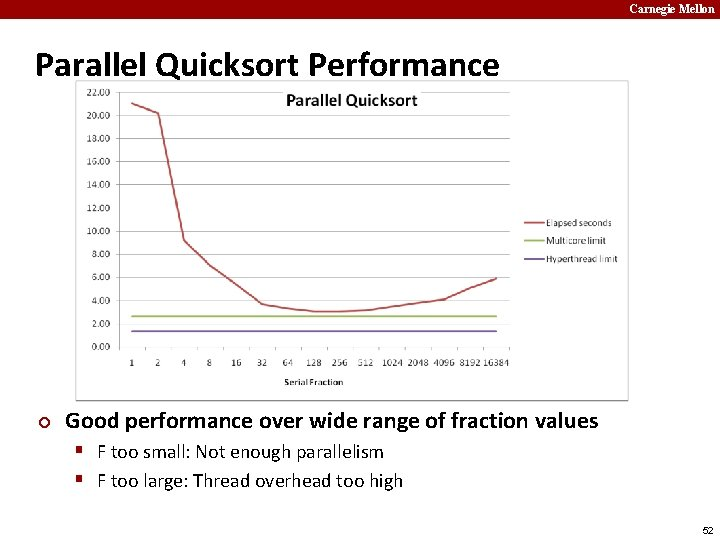Carnegie Mellon Parallel Quicksort Performance ¢ Good performance over wide range of fraction values § F too small: Not enough parallelism § F too large: Thread overhead too high 52Carnegie Mellon Amdahl’s Law ¢ Overall problem § T Total sequential time required § p Fraction of total that can be sped up (0 p 1) § k Speedup factor ¢ Resulting Performance § Tk = p. T/k + (1 -p)T Portion which can be sped up runs k times faster § Portion which cannot be sped up stays the same § Maximum possible speedup § k= § T = (1 -p)T § 53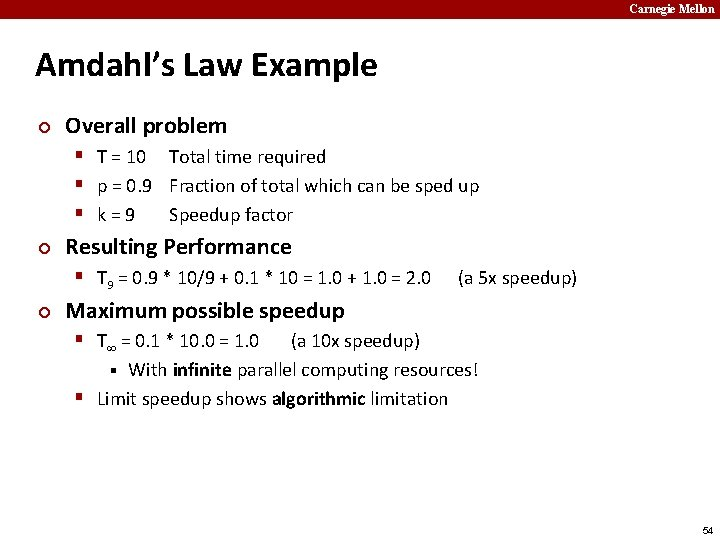Carnegie Mellon Amdahl’s Law Example ¢ Overall problem § T = 10 Total time required § p = 0. 9 Fraction of total which can be sped up § k=9 Speedup factor ¢ Resulting Performance § T 9 = 0. 9 * 10/9 + 0. 1 * 10 = 1. 0 + 1. 0 = 2. 0 ¢ (a 5 x speedup) Maximum possible speedup § T = 0. 1 * 10. 0 = 1. 0 (a 10 x speedup) § With infinite parallel computing resources! § Limit speedup shows algorithmic limitation 54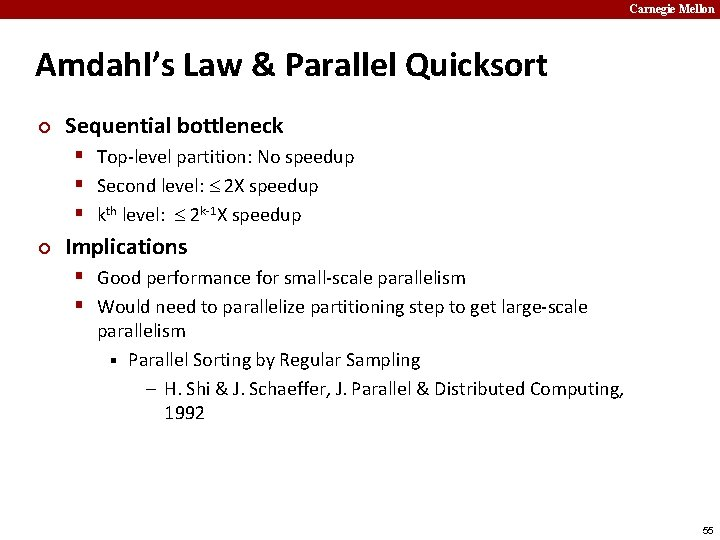Carnegie Mellon Amdahl’s Law & Parallel Quicksort ¢ Sequential bottleneck § Top-level partition: No speedup § Second level: 2 X speedup § kth level: 2 k-1 X speedup ¢ Implications § Good performance for small-scale parallelism § Would need to parallelize partitioning step to get large-scale parallelism § Parallel Sorting by Regular Sampling – H. Shi & J. Schaeffer, J. Parallel & Distributed Computing, 1992 55Carnegie Mellon Lessons Learned ¢ Must have parallelization strategy § Partition into K independent parts § Divide-and-conquer ¢ Inner loops must be synchronization free § Synchronization operations very expensive ¢ Watch out for hardware artifacts § Need to understand processor & memory structure § Sharing and false sharing of global data ¢ Beware of Amdahl’s Law § Serial code can become bottleneck ¢ You can do it! § Achieving modest levels of parallelism is not difficult § Set up experimental framework and test multiple strategies 58